# Finite Integration Technique

## What is FIT?

The Finite Integration Technique (FIT) is a consistent discretization scheme for Maxwell’s equations in their integral form.

The resulting matrix equations of the discretized fields can be used for efficient numerical simulations.

In addition, the basic algebraic properties of this discrete electromagnetic field theory allow to analytically and algebraically prove conservation properties with respect to energy and charge of the discrete formulation and gives an explanation of the stability properties of numerical formulations in the time domain.

So FIT is a numerical simulation method for approximation-free solutions of Maxwell's equations in their integral form. It is also the mathematical base for simulation software MAFIA and CST MICROWAVE STUDIO®.

## Basics

The Finite Integration Technique (FIT) was first presented in 1977 by Prof. Thomas Weiland (TU Darmstadt) . It provides a discrete reformulation of Maxwell’s equations in their integral form suitable for computers and it allows to simulate real-world electromagnetic field problems with complex geometries.

This finite volume-type discretization scheme for Maxwell’s equations is based upon the usage of integral balances and thus allows to prove stability and conservation properties of the discrete fields even before starting with numerical calculations, as described in .

Algebraic properties of the discrete formulation make it possible to develop long-term stable numerical time integration schemes or accurate eigenvalue solvers avoiding spurious modes.

In the resulting discrete formulations the equations are typically separated in those which are metric-free, arising from topology, and in those which are metric-depended. They closely resemble the discrete formulations of the Finite Integration Technique.

This mathematical background is corresponding with conformal Edge-Finite-Element schemes used in computational electromagnetics, which are usually rather derived starting from mathematical variational formulations.

The first discretization step of the FI-method consists in the restriction of the electromagnetic field problem, which usually represents an open boundary problem, to a simply connected and bounded space region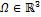, which contains the space region of interest.

The next step consists in the decomposition of the computational domain Ω into a locally finite number of simplicial cells Vi such as tetra- or hexahedra under the premise that all cells have to fit exactly to each other, i.e. the intersection of two different cells is either empty or it must be a two-dimensional polygon, a one-dimensional edge shared by both cells or a point. This  decomposition yields the finite simplicial cells complex G, which serves as computational grid.

This spatial discretization is a very generic cell-based approach and consequently, the FI-theory is not restricted to three-dimensional Cartesian meshes only; all types of coordinate meshes, orthogonal as well as non-orthogonal meshes can be considered. Also consistent subgrid schemes, which correspond to local mesh refinement including grid line termination techniques are already developed. The FI-Technique extends to non-simplicial cells, as long as the resulting cell complex is homeomorphic to a simplicial cell complex. For practical application such general cell complexes, where the cell edges may be curves, only play a role if they occur as coordinate meshes.

Each edge of the cells includes an initial orientation, of such manner that the union of these cell edges can be described as a directed graph. Also polygonal facets of the complex are associated with a direction.

For the sake of simplicity, it is assumed that Ω is brickshaped in the following description of the FI-technique and that the decomposition is given with a with a tensor product grid for Cartesian coordinates such that we get a cell complex G.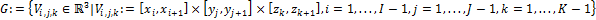The nodes (xi, yj, zk) are enumerated with the coordinates i, j and k along the x−, y− and z−axis. This results in the total number of Np := I · J ·K mesh points for (I − 1)· (J − 1) · (K − 1) mesh cells.

After the definition of the grid cell complex G, the further introduction of the FI-theory can be restricted to a single cell volume Vn. Starting with Faraday’s law in integral formcan be rewritten for a facet

Az(i, j, k) of Vn as the ordinary differential equation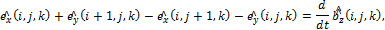The scalar value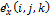is the electric voltage along one edge of the surface Az(i, j, k), representing the exact value of the integral over of the electric field along this edge. The scalar value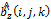represents the magnetic flux, i.e., the integral value over the magnetic flux density, through the cell facet Az(i, j, k). Note that the orientation of the cell edges will have influence on the signs within.

The integral formulation of Faraday’s law is valid for each single facet A(i, j, k) of G and the discrete approach naturally extends to larger facet areas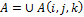. The same result will hold for surface integrals. This motivates the spatial discretization approach by a finite cell complex chosen within the Finite Integration Technique.

Assuming a lexicographical ordering of the electric voltagesand of the magnetic facet fluxesover the whole cell complex G and their assembly into column vectors in such a way, that we compose the degrees of freedom first in x−direction, then in y− and z−direction, we get two vectors e and b.

The equations of all grid cell surfaces of the complex G can be collected in a matrix form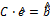.  The matrix C contains only topological information on the incidence relation of the cell edges within G and on their orientation, thus it only has matrix coefficients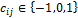. It represents a discrete curl-operator on the grid G.

References

 Thomas Weiland, “A discretization method for the solution of Maxwell’s equations for six-component fields,” Electronics and Communications AEU,  Vol. 31, No. 3, 116–120, 1977

 M. Clemens and T. Weiland, Discrete Electromagnetism with the Finite Integration Technique, Progress In Electromagnetics Research, PIER 32, 65–87, 2001

Author : Klaus Debes